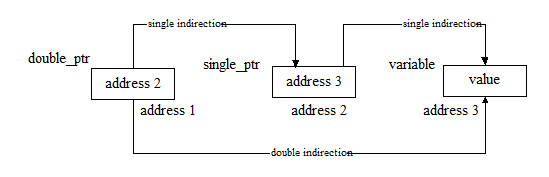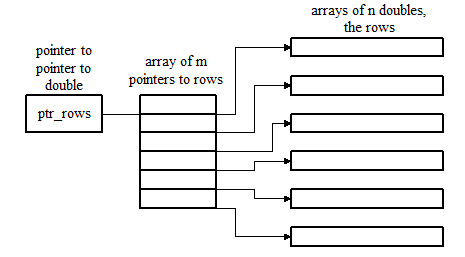## Pointers To Pointers Multiple Indirection In C Language

### Multiple Indirection - Pointers to Pointers:

It is possible in C to have a pointer point to another pointer that points to a target value. This is termed multiple indirection in this case double indirection. Multiple indirection can be carried out to whatever extent is desired but can get convoluted if carried to extremes.

In the normal situation, single indirection, a pointer variable would hold the address in memory of an appropriate variable, which could then be accessed indirectly by de-referencing the pointer using the * operator.

In the case of double indirection, we have the situation where a variable may be pointed to by a pointer as with single indirection, but that this pointer may also be pointed to by another pointer. So we have the situation where we must de-reference this latter pointer twice to actually access the variable we are interested in. De-referencing the pointer to a pointer once gives us a normal singly indirected pointer, de-referencing the pointer to a pointer secondly allows us to access the actual data variable. The situation is depicted in the diagram below.To declare a pointer to a pointer we include another indirection operator

Syntax
`float  * * ptr ;`

which in this case defines a pointer to a pointer to type float.

The following illustrates some valid operations using double indirection.

Syntax
```int x = 10, *p, **q ;

p = &x ;
q = &p ;

**q = 20 ;       // de-reference twice to access value
p = *q ;        // de-reference q once to get a pointer to int
…
int array1[] = { 1,2,3,4,5,6 ,7 ,8,9,10} ;
int array2[] = {10,20,30,40,50} ;
int *pointers ;            // an array of pointers to type int
int **ptr ;                // a doubly indirected pointer

ptr = pointers ;        // initialise pointer to array of pointers
*ptr++ = array1 ;    // now we simply de-reference the pointer to a pointer
*ptr = array2 ;        // once and move it on like any pointer

**ptr = 100 ;        // ptr is pointing at pointers which in turn is pointing
// at array2 so array2 is assigned 100```

For Example :- Allocation and initialisation of an m x n matrix using double indirection

What we require here is to allocate an n x n matrix as a collection of discrete rows rather than just as one block of memory. This format has advantages over a single block allocation in certain situations. The structure we end up with is illustrated below.CODE/PROGRAM/EXAMPLE
```#include <stdio.h>
#include <malloc.h>

void main( void )
{
double **ptr_rows, **user_ptr, *elem_ptr ;
int m, n, i, j ;

printf( “\n\nEnter the number of rows and columns required (m, n) : “ ) ;
scanf( “%d, %d”, &m, &n ) ;
_flushall() ;

ptr_rows = ( double **) malloc( m * sizeof ( double * ) ) ;        // space for row pointers

user_ptr = ptr_rows ;
for ( i = 0; i < m ; i++ )                        // and then row elements
{
*user_ptr = (double *) malloc( n * sizeof( double ) ) ;

elem_ptr = *user_ptr ;
for ( j = 0; j < n ; j++ )
*elem_ptr++ = 1.0 ;

user_ptr++ ;             // move onto next row pointer
}

// after use we need to clean up in reverse order
user_ptr = ptr_rows ;

for ( i = 0; i < n; i++ )
free( *user_ptr ++ ) ;        // free a row and move onto next

free( ptr_rows ) ;            // free pointers to rows
}```
#pointers_to_pointers_multiple_indirection_in_c_language #Multiple_Indirection_-_Pointers_to_Pointers #Pointers_-_Multiple_Indirection

### (New page will open, for Comment)

Not yet commented...